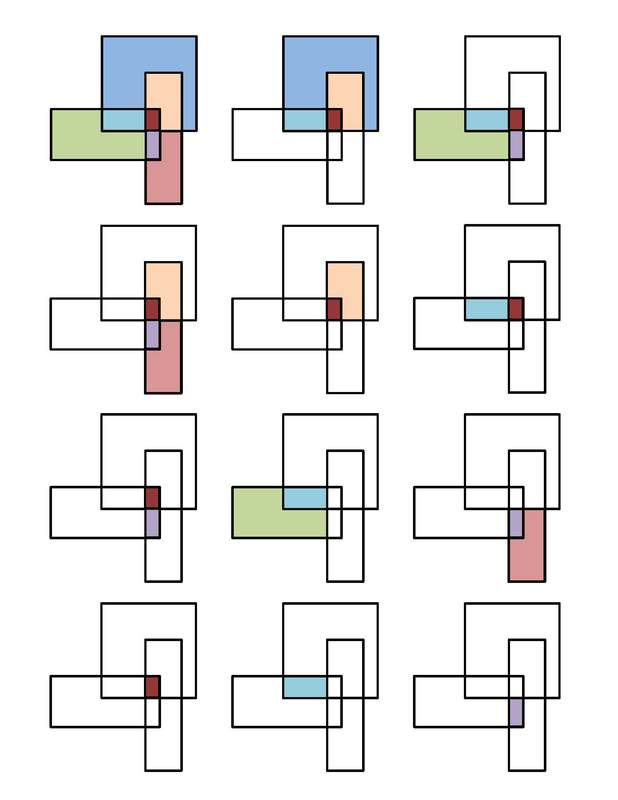# Overlapping Rectangles

Alignments to Content Standards: 1.G.A.2

How many rectangles are in this picture?## IM Commentary

The purpose of this task is to give students an opportunity to compose and decompose polygons to make rectangles. This is a challenging problem for first graders and it would be inappropriate to use it as an assessment. However, if presented as a brainteaser it can be useful for giving the students practice in recognizing rectangles, and stimulate interest as students compete to try to find the most rectangles. Furthermore, older students may also benefit from such an exercise as well, which could be aligned with 2.G.1 or even 3.G.1.

Students could work in teams and would benefit from having lots of copies of the figure and colored pencils at hand. Then students could compare their rectangles and sort them into piles which contain the same rectangles to help them decide if two rectangles found by different students are actually the same rectangle. This will help reduce the chances that a rectangle gets counted twice.

While inappropriate for assessment purposes in elementary school, this task was adapted from problem #19 on the 2011 American Mathematics Competition (AMC) 8 Test. For older students, the focus is not so much on understanding how to compose shapes from other shapes, but on finding a systematic way of enumerating the rectangles. The most efficient way to do this is to identify the seven regions in some way that is easy to refer to and check to see that all the ways of combining them that result in a rectangle are included in the list. An example of how this approach might look is in the third solution. This task shows how concepts that students learn in elementary school can reappear in more sophisticated forms in much later grades.

## Solutions

Solution: 1As shown, there are 11 rectangles.

The 11 rectangles in the first solution can be viewed as being composed of the non-overlapping regions shown here:The rectangles shown in the first solution, color coded by region, are shown below:Students who color the regions distinct colors are showing the decomposition called for in the standard more clearly, but as this is an exploratory challenge problem for 1st graders, it is not required that they do it this way.

Solution: 3

If this task were used with significantly older students, a more abstract representation of the rectangles might be accessible to them:

Partition the figure into non-overlapping regions as shown. The list of rectangles are $b$, $c$, $d$, $ab$, $bc$, $cd$, $cf$, $de$, $abcd$, $bcfg$, and $cdef$.